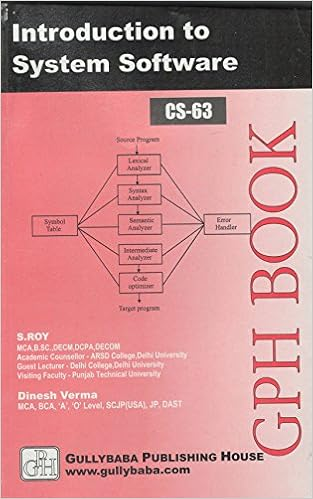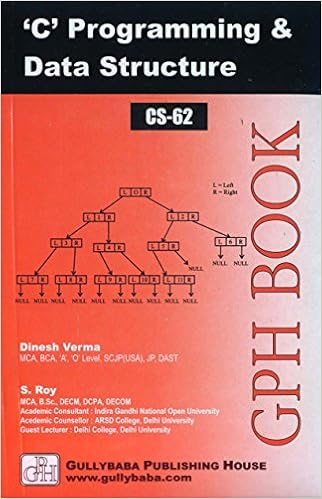IGNOU BCA MCA.comQuestion Papers of IGNOU MCA-OLD CS02

CS02: Introduction to Software
Year: 2003 TEE: December Time 2 Full Marks 60
Note: Question 1 is compulsory. Answer any three from the rest.

Q.1(a): Design an algorithm to compute 1/n! for a given n.

Q.1(b): Write a shell program to generate the first n terms of the following squence without using multiplication: 

Q.1(c): Consider the following set of process which arrive in the ready queue at the same time:

 Process CPU Time P1 3 P2 2 P3 4 P4 2
Consider the follwing scheduling algorithms: FCFS, SJF and Round Robin (quantum=1).
(i) What is the turnaround time of each process for each of the above sheduling algorithm?
(ii) What is the waiting time of each process for each of the avove algirithms?: 

Q.1(d): What is a 4GL ? How do 4GLs help to solve problem? Mention the merits and demerits of the usage of 4GLs in application development.: 

home || Next >>